## Design Patterns Using Advanced Chart Features

### Temporal Logic

Stateflow® temporal logic operators (such as `after`, `before`, or `every`) are Boolean operators that operate on recurrence counts of Stateflow events. Temporal logic operators can appear only in conditions on transitions that originate from states, and in state actions. Although temporal logic does not introduce new events into a Stateflow model, it is useful to think of the change of value of a temporal logic condition as an event. You can use temporal logic operators in many cases where a counter is required. A common use case would be to use temporal logic to implement a time-out counter.

For detailed information, see Control Chart Execution by Using Temporal Logic (Stateflow).

The chart shown in the following figure uses temporal logic in a design for a debouncer. Instead of instantaneously switching between on and off states, the chart uses two intermediate states and temporal logic to ignore transients. The transition is committed based on a time-out.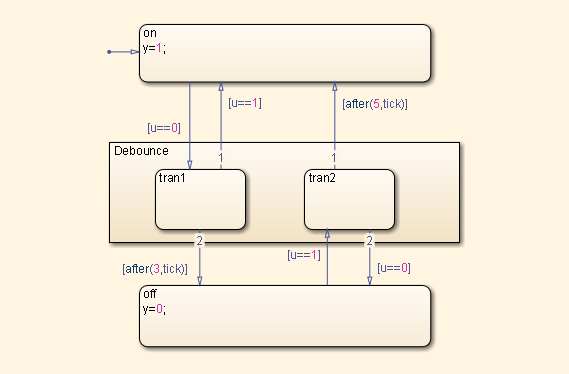By default, states in a Stateflow Chart are ordered alphabetically. The ordering of states in the HDL code can potentially vary if you enable active state output port generation in the HDL code. To enable this setting, open the Chart properties and select the Create output port for monitoring check box. See also Simplify Stateflow Charts by Incorporating Active State Output (Stateflow).

When you generate VHDL® code, the recently added state is selected as the `OTHERS` state in the HDL code. The following code excerpt shows VHDL code generated from this Chart.

```Chart_1_output : PROCESS (is_Chart, u, temporalCounter_i1, y_reg) VARIABLE temporalCounter_i1_temp : unsigned(7 DOWNTO 0); BEGIN temporalCounter_i1_temp := temporalCounter_i1; is_Chart_next <= is_Chart; y_reg_next <= y_reg; IF temporalCounter_i1 < 7 THEN temporalCounter_i1_temp := temporalCounter_i1 + 1; END IF; CASE is_Chart IS WHEN IN_tran1 => IF u = 1.0 THEN is_Chart_next <= IN_on; y_reg_next <= 1.0; ELSIF temporalCounter_i1_temp >= 3 THEN is_Chart_next <= IN_off; y_reg_next <= 0.0; END IF; WHEN IN_tran2 => IF temporalCounter_i1_temp >= 5 THEN is_Chart_next <= IN_on; y_reg_next <= 1.0; ELSIF u = 0.0 THEN is_Chart_next <= IN_off; y_reg_next <= 0.0; END IF; WHEN IN_off => IF u = 1.0 THEN is_Chart_next <= IN_tran2; temporalCounter_i1_temp := to_unsigned(0, 8); END IF; WHEN OTHERS => IF u = 0.0 THEN is_Chart_next <= IN_tran1; temporalCounter_i1_temp := to_unsigned(0, 8); END IF; END CASE; temporalCounter_i1_next <= temporalCounter_i1_temp; END PROCESS Chart_1_output;```

### Graphical Function

A graphical function is a function defined graphically by a flow diagram. Graphical functions reside in a chart along with the diagrams that invoke them. Like MATLAB® functions and C functions, graphical functions can accept arguments and return results. Graphical functions can be invoked in transition and state actions.

The following figure shows a graphical function that implements a 64–by–64 counter.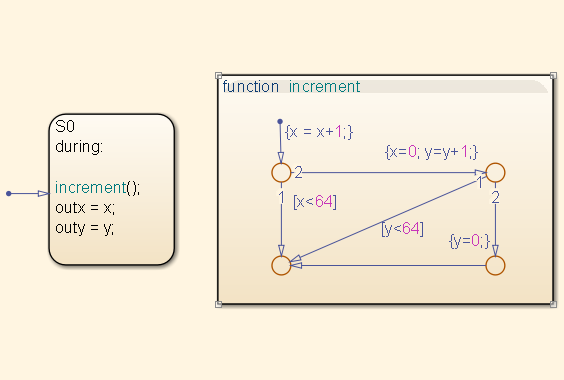The following code excerpt shows VHDL code generated for this graphical function.

``` x64_counter_sf : PROCESS (x, y, outx_reg, outy_reg) -- local variables VARIABLE x_temp : unsigned(7 DOWNTO 0); VARIABLE y_temp : unsigned(7 DOWNTO 0); BEGIN outx_reg_next <= outx_reg; outy_reg_next <= outy_reg; x_temp := x; y_temp := y; x_temp := tmw_to_unsigned(tmw_to_unsigned(tmw_to_unsigned(x_temp, 9), 10) + tmw_to_unsigned(to_unsigned(1, 9), 10), 8); IF x_temp < to_unsigned(64, 8) THEN NULL; ELSE x_temp := to_unsigned(0, 8); y_temp := tmw_to_unsigned(tmw_to_unsigned(tmw_to_unsigned(y_temp, 9), 10) + tmw_to_unsigned(to_unsigned(1, 9), 10), 8); IF y_temp < to_unsigned(64, 8) THEN NULL; ELSE y_temp := to_unsigned(0, 8); END IF; END IF; outx_reg_next <= x_temp; outy_reg_next <= y_temp; x_next <= x_temp; y_next <= y_temp; END PROCESS x64_counter_sf;```

### Hierarchy and Parallelism

Stateflow charts support both hierarchy (states containing other states) and parallelism (multiple states that can be active simultaneously).

In Stateflow semantics, parallelism is not synonymous with concurrency. Parallel states can be active simultaneously, but they are executed sequentially according to their execution order. (Execution order is displayed on the upper right corner of a parallel state).

For detailed information on hierarchy and parallelism, see Overview of Stateflow Objects (Stateflow) and Execution Order for Parallel States (Stateflow).

For HDL code generation, an entire chart maps to a single output computation process. Within the output computation process:

• The execution of parallel states proceeds sequentially.

• Nested hierarchical states map to nested `CASE` statements in the generated HDL code.

### Stateless Charts

Charts consisting of pure flow diagrams (i.e., charts without states ) are useful in capturing `if-then-else` constructs used in procedural languages like C.

As an example, consider the following logic, expressed in C-like pseudocode.

```if(U1==1) { if(U2==1) { Y = 1; }else{ Y = 2; } }else{ if(U2<2) { Y = 3; }else{ Y = 4; } } ```

The following figure shows the flow diagram that implements the `if-then-else` logic.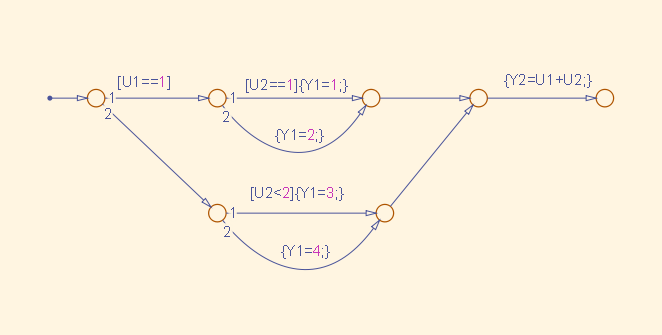The following generated VHDL code excerpt shows the nested IF-ELSE statements obtained from the flow diagram.

```Chart : PROCESS (Y1_reg, Y2_reg, U1, U2) -- local variables BEGIN Y1_reg_next <= Y1_reg; Y2_reg_next <= Y2_reg; IF unsigned(U1) = to_unsigned(1, 8) THEN IF unsigned(U2) = to_unsigned(1, 8) THEN Y1_reg_next <= to_unsigned(1, 8); ELSE Y1_reg_next <= to_unsigned(2, 8); END IF; ELSIF unsigned(U2) < to_unsigned(2, 8) THEN Y1_reg_next <= to_unsigned(3, 8); ELSE Y1_reg_next <= to_unsigned(4, 8); END IF; Y2_reg_next <= tmw_to_unsigned(tmw_to_unsigned(tmw_to_unsigned(unsigned(U1), 9),10) + tmw_to_unsigned(tmw_to_unsigned(unsigned(U2), 9), 10), 8); END PROCESS Chart;```

### Truth Tables

HDL Coder™ supports HDL code generation for:

• Truth Table functions within a Stateflow chart

• Truth Table (Stateflow) blocks in Simulink® models

This section examines a Truth Table function in a chart, and the VHDL code generated for the chart.

Truth Tables are well-suited for implementing compact combinatorial logic. A typical application for Truth Tables is to implement nonlinear quantization or threshold logic. Consider the following logic:

```Y = 1 when 0 <= U <= 10 Y = 2 when 10 < U <= 17 Y = 3 when 17 < U <= 45 Y = 4 when 45 < U <= 52 Y = 5 when 52 < U ```

A stateless chart with a single call to a Truth Table function can represent this logic succinctly.

The following figure shows the `quantizer` chart, containing the Truth Table.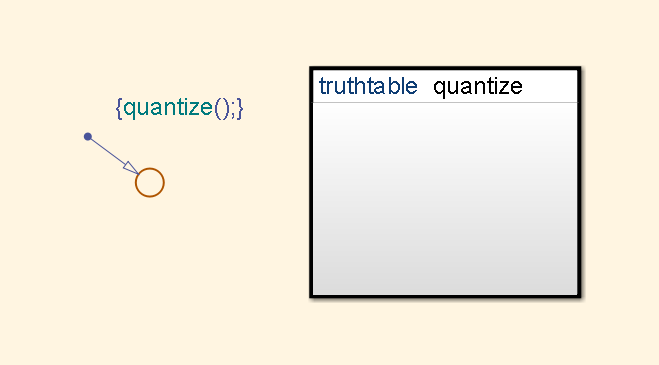The following figure shows the threshold logic, as displayed in the Truth Table Editor.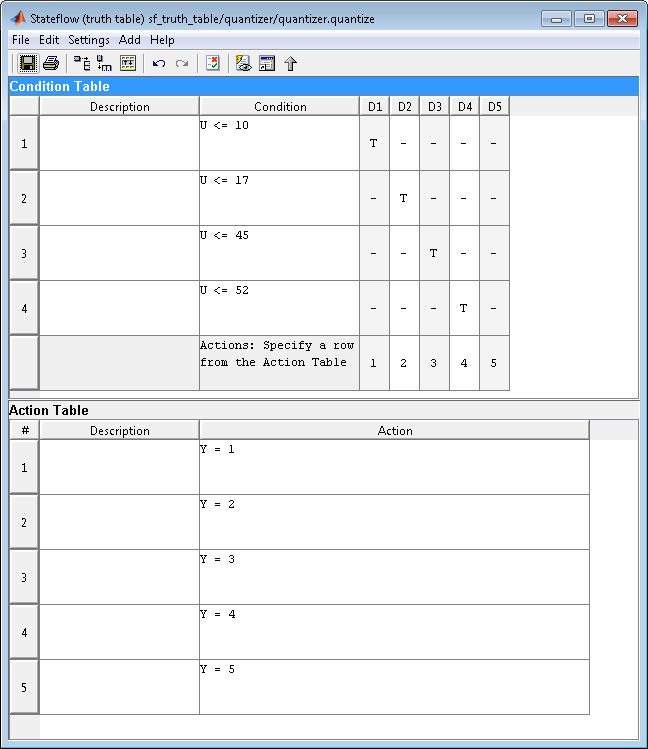The following code excerpt shows VHDL code generated for the `quantizer` chart.

``` quantizer : PROCESS (Y_reg, U) -- local variables VARIABLE aVarTruthTableCondition_1 : std_logic; VARIABLE aVarTruthTableCondition_2 : std_logic; VARIABLE aVarTruthTableCondition_3 : std_logic; VARIABLE aVarTruthTableCondition_4 : std_logic; BEGIN Y_reg_next <= Y_reg; -- Condition #1 aVarTruthTableCondition_1 := tmw_to_stdlogic(unsigned(U) <= to_unsigned(10, 8)); -- Condition #2 aVarTruthTableCondition_2 := tmw_to_stdlogic(unsigned(U) <= to_unsigned(17, 8)); -- Condition #3 aVarTruthTableCondition_3 := tmw_to_stdlogic(unsigned(U) <= to_unsigned(45, 8)); -- Condition #4 aVarTruthTableCondition_4 := tmw_to_stdlogic(unsigned(U) <= to_unsigned(52, 8)); IF tmw_to_boolean(aVarTruthTableCondition_1) THEN -- D1 -- Action 1 Y_reg_next <= to_unsigned(1, 8); ELSIF tmw_to_boolean(aVarTruthTableCondition_2) THEN -- D2 -- Action 2 Y_reg_next <= to_unsigned(2, 8); ELSIF tmw_to_boolean(aVarTruthTableCondition_3) THEN -- D3 -- Action 3 Y_reg_next <= to_unsigned(3, 8); ELSIF tmw_to_boolean(aVarTruthTableCondition_4) THEN -- D4 -- Action 4 Y_reg_next <= to_unsigned(4, 8); ELSE -- Default -- Action 5 Y_reg_next <= to_unsigned(5, 8); END IF; END PROCESS quantizer;```

Note

When generating code for a Truth Table (Stateflow) block in a Simulink model, HDL Coder writes a separate entity/architecture file for the Truth Table code. The file is named `Truth_Table.vhd` (for VHDL) or `Truth_Table.v` (for Verilog®).